Math resources Algebra

Inequalities

# Inequalities

Here you will learn about inequalities, including comparing quantities using inequalities, interpreting inequalities, representing inequalities, and solving inequalities.

Students first learn about inequality symbols in the first grade to represent relationships as part of their work with number and operations in base ten. They will expand upon this knowledge as they progress through elementary school into middle school, where they work with expressions and equations.

## What are inequalities?

Inequalities are a comparison between two numbers, values, or expressions.

One of the quantities may be less than, greater than, less than or equal to, or greater than or equal to the other things.

This image describes the shape and direction of the symbols.

When stacking identical blocks, the height of 3 blocks is greater than the height of 1 block. The lines joining the stacks give the shape and direction of the inequality symbols.

The symbols used to make the comparisons are in the table below.

\hspace{1.6cm} Comparison Symbols

Less than

Greater than

Less than or equal to

Greater than or equal to

Equal

Not equal

Step by step guide: Great than signs

Step by step guide: Less than signs

• You can use inequalities to make comparisons between quantities.

3 tens and 3 ones \hspace{2cm} 4 tens and 1 ones

\bf{33} \hspace{1.6cm}\textbf{<} \hspace{1.6cm} \bf{41} \hspace{0.5cm}

of 5 equal parts \hspace{2.5cm} of 5 equal parts

\bf{\cfrac{3}{5}} \hspace{1.8cm}\textbf{>} \hspace{1.9cm} \bf{\cfrac{2}{5}} \hspace{0.3cm}

40 \%<\cfrac{3}{5}

40 \%=\cfrac{40}{100}=\cfrac{40 \div 20}{100 \div 20}=\cfrac{2}{5}

\cfrac{2}{5}<\cfrac{3}{5}

• You can represent inequalities on a number line.

Recall, that x = 5 is an equation. The variable x is only equal to 5. In an equality, the value of x can be many numbers.

You can interpret and represent inequality statements on the number line.

An open circle shows it does not include the value.

A closed circle shows it does include the value.

\bf{\textbf{x} < 5}

Read as ‘x is less than 5’

The solution set is the numbers less
than 5.

\bf{\textbf{x} \leq 5}

Read as ‘x is less than or equal to 5’

The solution set is the numbers less
than or equal to 5.

\bf{\textbf{x} > 5}

Read as ‘x is greater than 5’

The solution set is all the numbers greater
than 5.

\bf{\textbf{x} \geq 5}

Read as ‘x is greater than or equal to 5’

The solution set is all the numbers greater
than or equal to 5.

• You can solve simple inequalities.

\begin{aligned} x+4&>9 \\ \colorbox{#dcd0ff}{-4} &\quad \colorbox{#dcd0ff}{-4} \\ x+4-4&>9-4 \\ x&>5 \end{aligned}

Represent the answer on the number line.

The solution is: x is any number greater
than 5.

\begin{aligned} x-8&\geq -2 \\ \colorbox{#dcd0ff}{+8} &\quad \colorbox{#dcd0ff}{+8} \\ x-8+8&\geq -2+8 \\ x&\geq 6 \end{aligned}

Represent the answer on the number line.

The solution is: x is any number greater
than 6 or equal to 6.

\begin{aligned} 3x&<12 \\ \cfrac{3x}{3}&<\cfrac{12}{3} \\ x&<4 \end{aligned}

Represent the answer on the number line.

The solution is: x is any number lesser
than 4.

\begin{aligned} \cfrac{x}{-2} \, &\leq -2 \\ \colorbox{#dcd0ff}{× −2} &\quad \colorbox{#dcd0ff}{× −2} \\ x&\geq 4 \end{aligned}

(flip the inequality symbol when multiplying
by a negative number)

The solution is: x is any number greater
than or equal to 4.

### What are inequalities?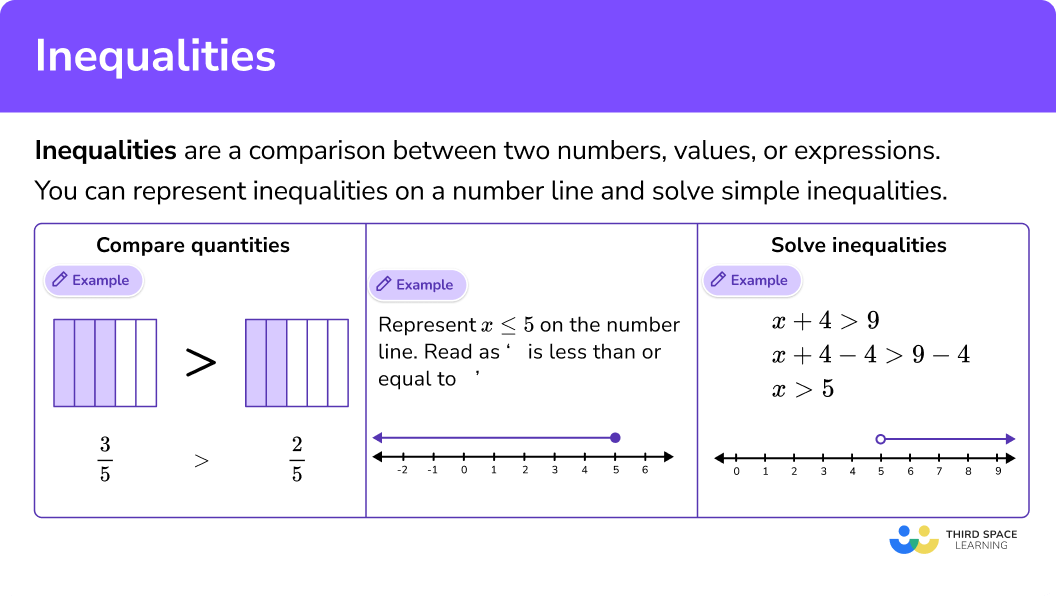## Common Core State Standards

How does this relate to 1st through 6th grade math?

• Grade 1 – Number and Operations Base 10 (1.NBT.B.3)
Compare two two-digit numbers based on meanings of the tens and ones digits, recording the results of comparisons with the symbols >, \, =, and <.

• Grade 2 – Number and Operations Base 10 (2.NBT.A.4)
Compare two three-digit numbers based on meanings of the hundreds, tens, and ones digits, using >, \, =, and < symbols to record the results of comparisons.

• Grade 3 – Number and Operations – Fractions (3.NF.A.3.d)
Compare two fractions with the same numerator or the same denominator by reasoning about their size. Recognize that comparisons are valid only when the two fractions refer to the same whole.
Record the results of comparisons with the symbols >, \, =, or <, and justify the conclusions, for example, by using a visual fraction model.

• Grade 4 – Number and Operations Base Ten (4.NBT.A.2)
Read and write multi-digit whole numbers using base-ten numerals, number names, and expanded form. Compare two multi-digit numbers based on meanings of the digits in each place, using >, \, =, and < symbols to record the results of comparisons.

• Grade 4 – Number and Operations – Fractions (4.NF.A.2)
Compare two fractions with different numerators and different denominators, for example by creating common denominators or numerators, or by comparing to a benchmark fraction such as \frac{1}{2}.
Recognize that comparisons are valid only when the two fractions refer to the same whole. Record the results of comparisons with symbols >, \, =, or <, and justify the conclusions, for example, by using a visual fraction model.

• Grade 4 – Number and Operations – Fractions (4.NF.C.7)
Compare two decimals to hundredths by reasoning about their size. Recognize that comparisons are valid only when the two decimals refer to the same whole. Record the results of comparisons with the symbols >, \, =, or <, and justify the conclusions, for example, by using a visual model.

• Grade 5 – Number and Operations Base Ten (5.NBT.A.3b)
Compare two decimals to thousandths based on meanings of the digits in each place, using >, \, =, and < symbols to record the results of comparisons.

• Grade 6 – Number System (6.NS.C.7.a)
Interpret statements of inequality as statements about the relative position of two numbers on a number line diagram. For example, interpret –3 > –7 as a statement that –3 is located to the right of –7 on a number line oriented from left to right.

• Grade 6Expressions and Equations (6.EE.B.8)
Write an inequality of the form x > c or x < c to represent a constraint or condition in a real-world or mathematical problem. Recognize that inequalities of the form x > c or x < c have infinitely many solutions; represent solutions of such inequalities on number line diagrams.

• Grade 6Expressions and Equations (6.EE.B.5)
Understand solving an equation or inequality as a process of answering a question: which values from a specified set, if any, make the equation or inequality true? Use substitution to determine whether a given number in a specified set makes an equation or inequality true.

• Grade 7 – Expressions and Equations (7.EE.4b)
Solve word problems leading to inequalities of the form px + q > r or px + q < r, where p, q, and r are specific rational numbers. Graph the solution set of the inequality and interpret it in the context of the problem.
For example: As a salesperson, you are paid \$50 per week plus \$3 per sale. This week you want your pay to be at least \100. Write an inequality for the number of sales you need to make, and describe the solutions. ## How to compare quantities using inequalities In order to compare quantities using inequalities: 1. Choose a form to represent the quantities. 2. Compare the quantities. 3. Write the comparison using the appropriate inequality symbol Step by step guide: Converting fractions, decimals and percentages ## How to graph inequalities on a number line In order to graph inequalities on a number line: 1. Identify the starting number. 2. Place a closed circle or open circle on the number. 3. Draw a line from the number in the direction representing the inequality. ## How to solve inequalities In order to solve one step inequalities: 1. Choose one side of the inequality to have the variable alone. 2. Use the additive inverse or multiplicative inverse to get the variable alone. 3. Write your solution with the inequality symbol. 4. Graph the solution set on the number line. Step by step guide: Solving inequalities ## Inequalities examples ### Example 1: compare fractions using an inequality Compare the fractions using a <, \, >, \, =. 1. Choose a form to represent the quantities. Both numbers are in the same form, so leave them as fractions. Get a common denominator. The common denominator between \, \cfrac{2}{3} \, and \, \cfrac{1}{4} is 12. \begin{aligned} &\cfrac{2}{3}=\cfrac{2 \, \times \, 4}{3 \, \times \, 4}=\cfrac{8}{12}\\\\ &\cfrac{1}{4}=\cfrac{1 \, \times \, 3}{4 \, \times \, 3}=\cfrac{3}{12} \end{aligned} 2Compare the quantities. \cfrac{8}{12} \, is greater than \, \cfrac{3}{12} 3Write the comparison using the appropriate inequality symbol. \cfrac{8}{12} \, > \, \cfrac{3}{12} So, \cfrac{2}{3} \, > \, \cfrac{1}{4} For a detailed explanation, go to: Step by step guide: Comparing Fractions ### Example 2: compare percents and fractions using inequalities Compare the fractions using a <, \, >, \, =. Choose a form to represent the quantities. Compare the quantities. Write the comparison using the appropriate inequality symbol. ### Example 3: represent an inequality on the number line Represent x \geq-1 Identify the starting number. Place a closed circle or open circle on the number. Draw a line from the starting number in the direction representing the inequality. Step-by-step guide: Inequalities on a number line ### Example 4: solve the inequality Solve the inequality: x+5 \leq-8 Choose one side of the inequality to have the variable alone. Use the additive inverse or multiplicative inverse to get the variable alone. Write your solution with the inequality symbol. Graph the solution set on the number line. ### Example 5: solve the inequality Solve the inequality: -4x < -16 Choose one side of the inequality to have the variable alone. Use the additive inverse or multiplicative inverse to get the variable alone. Write your solution with the inequality symbol. Graph the solution set on the number line. ### Example 6: real world application of inequalities Julie spends at least \10.25 on each movie ticket. Write and graph an inequality representing this.

Identify the starting value.

Decide if the starting value is included in the solution set.

Identify the solution set with a straight line.

### Teaching tips for inequalities

• To help students memorize the inequality symbols, make sure they are posted in the classroom or somewhere else easily accessible. Also encourage students to read inequalities both forward and backwards, which forces them to focus on the shape of the inequality symbol.

• When introducing the and ≥, explicitly make the connection between these signs and the greater than, less than, and equal sign.

• Number line worksheets are a good way to help students develop this skill, but be sure to also include activities that are more interactive. This could include graphing inequalities digitally or using things that happen in and around the classroom as a basis for writing and graphing real world inequalities.

• Demonstrate to students using the number line why the inequality symbol flips when multiplying or dividing by a negative number.

• Reinforce conceptual understanding using a number line between inequalities and equalities.

### Easy mistakes to make

• Confusing the inequality symbols
For example, comparing 5 < 4 and reading it as “5 is greater than 4” .

• Forgetting to flip the inequality symbol when dividing or multiplying by a negative number when solving the inequality
For example,

• Reading the inequality incorrectly from a number line
For example,
Reading this number line as x > 4 instead of x ≥ 4

### Practice inequalities questions

1. Choose the correct symbol to make the comparison.\le<>\geCompare the fractions by finding the common denominator and use equivalent fractions to change the fractions.

The common denominator is 14.

\begin{aligned} & \cfrac{2}{7}=\cfrac{2 \, \times \, 2}{7 \, \times \, 2}=\cfrac{4}{14} \\\\ & \cfrac{1}{2}=\cfrac{1 \, \times \, 7}{2 \, \times \, 7}=\cfrac{7}{14} \\\\ & \cfrac{4}{14} \, < \, \cfrac{7}{14} \end{aligned}

2. Choose the graph that represents the inequality.

x \ge-1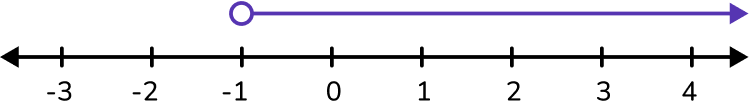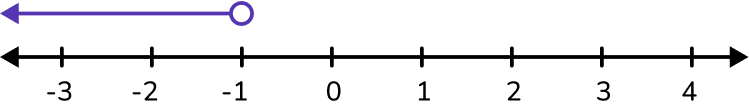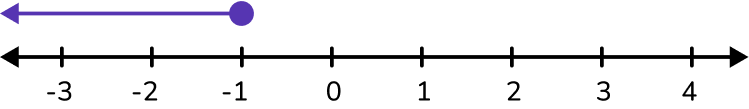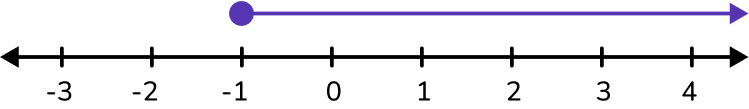x \ge-1 is read as ‘x is greater than or equal to -1’

-1 is in the solution set so the circle is closed. The arrow points towards the right because that represents the numbers greater than.3. Solve the inequality:

x-7 < 4

x > 11x \le 11x < 11x \ge 11Keep the variable on the left side of the inequality symbol. Use the additive inverse to solve the inequality.

The additive inverse of -7 is + 7 because -7 + 7 = 0. In other words, add 7 to both sides of the inequality.

\begin{aligned} & x-7<4 \\\\ & x-7+7<4+7 \\\\ & x<11 \end{aligned}

4. Solve the inequality:

5 x \geq 20

x < 4x \le 4x > 4x \ge 4Keep the variable on the left side of the inequality symbol. Use the multiplicative inverse to solve the inequality.

The multiplicative inverse is 5 \times \cfrac{1}{5}=1. In other words, divide each side of the inequality by 5.

\begin{aligned} & 5 x>20 \\\\ & \cfrac{5 x}{5} > \cfrac{20}{5} \\\\ & x>4 \end{aligned}

5. Which number line represents the solution set to the following inequality?

x + 3 < 4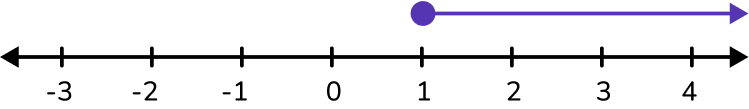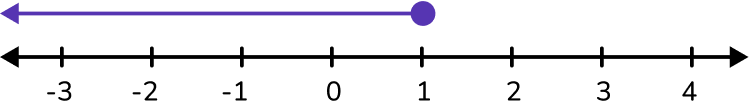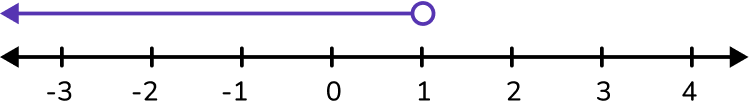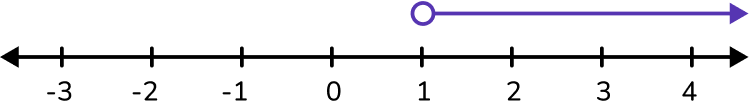Keep the variable on the left side of the inequality symbol. Use the additive inverse to solve the inequality.

The additive inverse of +3 is -3 because 3-3 = 0. In other words, subtract 3 from both sides of the inequality.

\begin{aligned} & x+3<4 \\\\ & x+3-3<4-3 \\\\ & x<1 \end{aligned}

6. Two times a number is greater than 6. Find the solution set.

x > 3x < 3x \geq 3x \leq 3Translate the problem into an inequality.

2 x>6

Solve the inequality. Keep the variable on the left side of the inequality symbol.

Use the multiplicative inverse to solve.

\begin{aligned} & 2 x>6 \\\\ & \cfrac{2 x}{2}>\cfrac{6}{2} \\\\ & x>3 \end{aligned}

## Inequalities FAQs

Can you graph more than one inequality on a number line?

Yes, when you get into pre-algebra and algebra you will be graphing compound inequalities on the number line which is more than one inequality.

Is moving to the right always the greater than direction and moving left the less than direction?

Yes, looking at a number line, right is a positive or greater than direction and left is a negative or less than direction.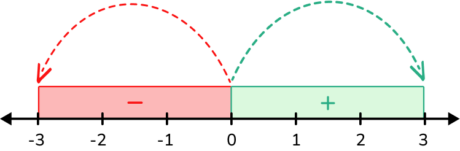Do all inequalities only have one variable?

No, just like expressions and equations, inequalities increasingly get more complex as students advance in their math learning. In 7th grade, students will continue learning by working with linear inequalities, also known as two-step inequalities.

Why do you flip the inequality symbol when multiplying or dividing by a negative number?

The inequality symbol flips because you have to keep the inequality as a true comparison. For example, if you have 3 < 5 and you multiply both sides of the inequality by -1 you will get -3 < -5. This is no longer a true inequality comparison. In order to make it true, you will have to flip the inequality symbol, so < becomes >.

-3 < -5 becomes -3 > -5.

Is solving linear inequalities the same as solving linear equations?

Yes, the way you would solve a linear inequality follows the same process as you would do to solve a linear equation. With equations, you choose a side of the equation to bring the variable to, and you would do the same for an inequality. It’s most common to have the variable on the left hand side of an equality and the integers or real numbers on the right side.

Are there other ways to express inequalities or inequality signs?

Yes, when you get into high school math, you can use interval notation with parentheses to represent inequalities.

Are there only linear inequalities?

No, there are other types of inequalities, such as quadratic and polynomial inequalities and absolute value inequalities, that you will represent and solve in high school math.

## Still stuck?

At Third Space Learning, we specialize in helping teachers and school leaders to provide personalized math support for more of their students through high-quality, online one-on-one math tutoring delivered by subject experts.

Each week, our tutors support thousands of students who are at risk of not meeting their grade-level expectations, and help accelerate their progress and boost their confidence.